# John Tranter

•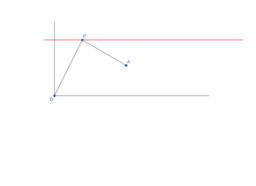### Q412

Activity

John Tranter

•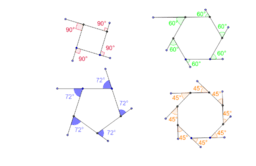### Exterior angles of a regular polygon

Activity

John Tranter

•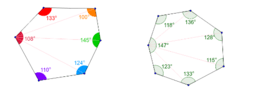### The interior angle sum of a polygon

Activity

John Tranter

•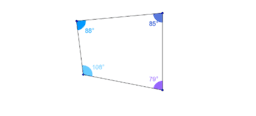Activity

John Tranter

•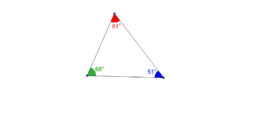### Angles in a triangle add up to 180°

Activity

John Tranter

•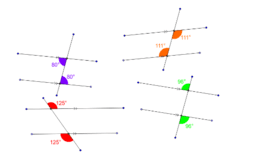### Alternate angles are equal

Activity

John Tranter

•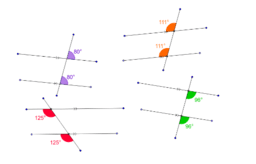### Corresponding angles are equal

Activity

John Tranter

•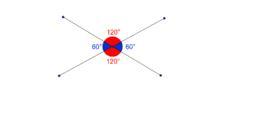### Vertically opposite angles are equal

Activity

John Tranter

•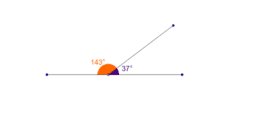### Angles on a straight line add up to 180°

Activity

John Tranter

•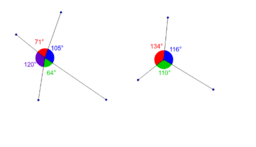### Angles at a point add up to 360°

Activity

John Tranter

•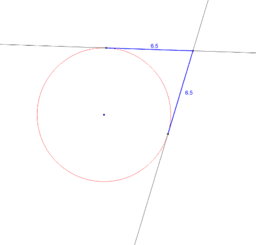### Tangents from an external point are equal in length.

Activity

John Tranter

•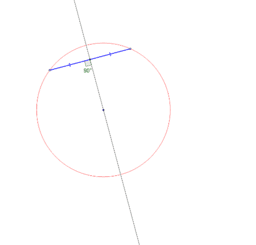### The the perpendicular bisector of a chord

Activity

John Tranter

•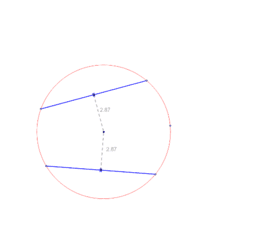### Equal chords are equidistant from the centre

Activity

John Tranter

•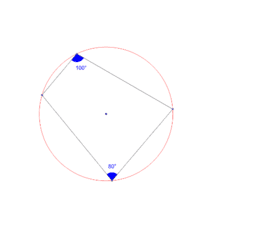### Opposite angles of a cyclic quadrilateral

Activity

John Tranter

•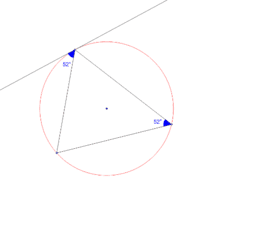### Angle between the tangent and a chord

Activity

John Tranter

•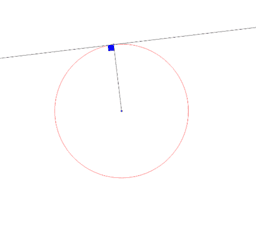### Angle between the tangent and the radius

Activity

John Tranter

•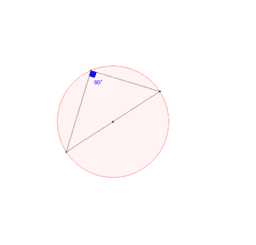### The angle in a semi-circle is a right angle

Activity

John Tranter

•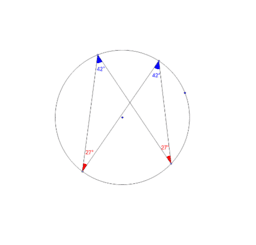### The angles in the same segment are equal

Activity

John Tranter

•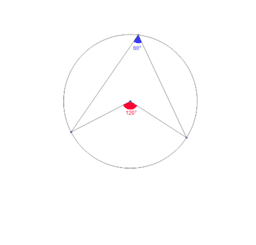Activity

John Tranter